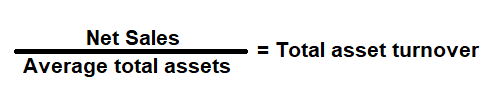# Asset Turnover Ratio Calculator

Key in the Net Sales and Total Assets Values to the respective fields given below and then click Calculate to get the desired result.

# Asset Turnover Ratio Calculator - Glossary:

Asset Turnover Ratio: Shows the proportion of Net Sales to its Average Total Assets. In simple words, it measures the efficiency of a company’s ability to use its asset to generate sales.

### Formula:### How to use this equation?

The values are commonly stated against total assets in the balance and net sales in the income statement. To use this ratio, divide net sales by its average total assets.
Note:
Average Total Assets: Add total assets of the current year and the total assets of the previous year, and then divide that value by 2.

Net Sales:
Total sales less returns and bad debts.

Total Assets:
Total assets are the sum of all current and noncurrent assets that a company owns.

Example:
Asset turnover ratio for a company with a net income of \$500,000 and average total assets of \$775,000 is 0.65:1. It means that for every dollar in assets, a company generates \$0.65 in sales.